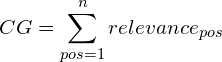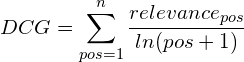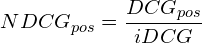# Measuring Search Relevance using NDCGNormalized Discounted Cumulative Gain (NDCG) is popular method for measuring the quality of a set of search results. It asserts the following:

1. Very relevant results are more useful than somewhat relevant results which are more useful than irrelevant results (cumulative gain)
2. Relevant results are more useful when they appear earlier in the set of results (discounting).
3. The result of the ranking should be irrelevant to the query performed (normalization).

Cumulative Gain (CG) is the predecessor of DCG and does not include the position of a result in the consideration of the usefulness of a result set. In this way, it is the sum of the graded relevance values of all results in a search result list. Suppose you were presented with a set of search results for a query and asked to rank each result:
0 => Not relevant 1 => Near relevant 2 => Relevant.

If we sum the values for a page of results we will have a measure of the cumulative gain (CG).Cumulative gain, however, doesn’t reward relevant results that appear higher in the result set. To achieve the Discounted cumulative gain (DCG) we must discount results that appear lower. The premise of DCG is that highly relevant documents appearing lower in a search result list should be penalized as the graded relevance value is reduced logarithmically proportional to the position of the result. A common method for doing this is to, effectively, divide by the natural log of the position:The final stage of the NDCG is normalization. If you calculate DCG for different queries you’ll find that some queries are just harder than others and will produce lower DCG scores than easier queries. Normalization solves this problem by scaling the results based off of the best result seen (called the ideal DCG or iDCG).The fact that you must determine the global iDCG before computing the NDCG for any given result makes implementation a bit tricky because you must first calculate the DCG for all results to determine the ideal value and then use it to calculate NDCG for each of the results.

Hope you found this useful.